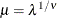The COUNTREG Procedure

MODEL Statement

Subsections:
• MODEL dependent-variable = <regressors> </ options>;

The MODEL statement specifies the dependent-variable and independent covariates (regressors) for the regression model. If you specify no regressors, PROC COUNTREG fits a model that contains only an intercept. The dependent count variable should take on only nonnegative integer values in the input data set. PROC COUNTREG rounds any positive noninteger count values to the nearest integer. PROC COUNTREG ignores any observations that have a negative count.

Only one MODEL statement can be specified. You can specify following options in the MODEL statement after a slash (/).

DIST=value

specifies a type of model to be analyzed. If you specify this option in both the MODEL statement and the PROC COUNTREG statement, then only the value in the MODEL statement is used. The following model types are supported:

POISSON | P

specifies a Poisson regression model.

CMPOISSON | C | CMP

specifies a Conway-Maxwell-Poisson regression model.

NEGBIN(P=1)

specifies a negative binomial regression model with a linear variance function.

NEGBIN(P=2) | NEGBIN

specifies a negative binomial regression model with a quadratic variance function.

ZIPOISSON | ZIP

specifies a zero-inflated Poisson regression. The ZEROMODEL statement must be specified when this model type is specified.

ZICMPOISSON | ZICMP

specifies a zero-inflated Conway-Maxwell-Poisson regression. The ZEROMODEL statement must be specified when this model type is specified.

ZINEGBIN | ZINB

specifies a zero-inflated negative binomial regression. The ZEROMODEL statement must be specified when this model type is specified.

ERRORCOMP=value

specifies a type of conditional panel model to be analyzed. The following model types are supported:

FIXED

specifies a fixed-effect error component regression model.

RANDOM

specifies a random-effect error component regression model.

NOINT

suppresses the intercept parameter.

OFFSET=variable

specifies a variable in the input data set to be used as an offset variable. The offset variable appears as a covariate in the model with its parameter restricted to 1. The offset variable cannot be the response variable, the zero-inflation offset variable (if any), or one of the explanatory variables. The "Model Fit Summary" table gives the name of the data set variable used as the offset variable; it is labeled as "Offset."

PARAMETER=MU | LAMBDA

specifies the parameterization for the Conway-Maxwell-Poisson model. The following parameterizations are supported:

LAMBDA

estimates the original Conway-Maxwell-Poisson model (Shmueli et al. (2005))

MU

reparameterizesas documented by Guikema and Coffelt (2008), whereand the integral part ofrepresents the mode, which can be considered a measure of central tendency (mean).

By default, PARAMETER=MU.

SELECT=INFO<(options)>
SELECTVAR=INFO<(options)>
SELECT=PEN<(options)>
SELECTVAR=PEN<(options)>

specifies variable selection.

SELECT=INFO requests that the variable selection method be based on an information criterion. For more information, see the section Variable Selection Using an Information Criterion. You can specify the following options:

DIRECTION=FORWARD | BACKWARD

specifies the search algorithm to use in the variable selection method. By default, DIRECTION=FORWARD.

CRITER=AIC | SBC

specifies the information criteria to use in the variable selection. By default, CRITER=SBC.

MAXSTEPS=value

specifies the maximum number of steps to allow in the search algorithm. The default is infinite; that is, the algorithm does not stop until the stopping criterion is satisfied.

LSTOP=value

specifies the stopping criterion. The value represents the percentage of decrease or increase in the AIC or SBC that is required for the algorithm to proceed; it must be a positive number less than 1. By default, LSTOP=0.

RETAIN(variable1 <variable2...>)

specifies that the variables named within parentheses be retained during the variable selection process.

SELECT=PEN requests the penalized likelihood variable selection method. For more information, see the section Variable Selection Using Penalized Likelihood. You can specify the following options:

LLASTEPS=value

specifies the maximum number of iterations in the algorithm of local linear approximations. By default, LLASTEPS=5.

GCVLENGTH=value

specifies the number of different values to use for the generalized cross validation (GCV) tuning parameter. The value corresponds toin the computations that are described in the section Variable Selection Using Penalized Likelihood. By default, GCVLENGTH=20.

GCV

specifies the generalized cross-validation (GCV) approach. For more information, see the section The GCV Approach.

GCV1

specifies the GCV1 approach. For more information, see the section The GCV1 Approach.

When SELECT=PEN, GCV1 is the default.

Printing Options

CORRB

prints the correlation matrix of the parameter estimates. The CORRB option can also be specified in the PROC COUNTREG statement.

COVB

prints the covariance matrix of the parameter estimates. The COVB can also be specified in the PROC COUNTREG statement.

ITPRINT

prints the objective function and parameter estimates at each iteration. The objective function is the negative log-likelihood function. The ITPRINT option can also be specified in the PROC COUNTREG statement.

PRINTALL

requests all printing options. The PRINTALL option can also be specified in the PROC COUNTREG statement.